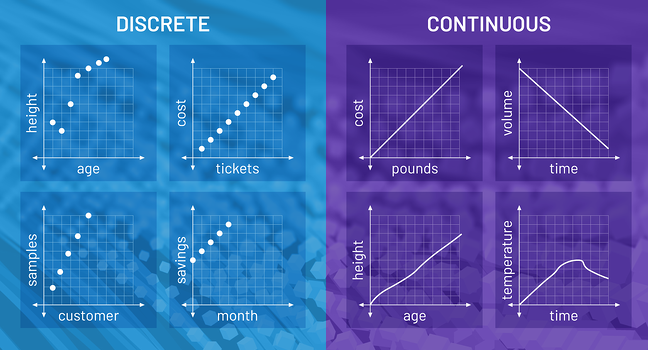top of page
Search

# 5Ws and 1Hs of a Regression Algorithm

#### What is regression?

Variables come in 2 categories, independent and dependent variables. Independent variables are the variables that we manipulate its values, while dependent variables are the variables whose value is affected by the change in independent variables. Regression is a machine learning technique that tries to uncover the relationship between these two types of variables.

#### How do regression models work?

There many types of regression models, here I will briefly describe the intricacies of the most common ones.

• Simple Linear Regression

The simplest out of all regression models. Data is scattered on a graph where the axes of it are the dependent and independent variables. The model will see this and attempt to find and plot a straight line across the data points. The line represents the relationship between the two variables and is then referenced for new data.A few things to note, this method is used only to find the relationship between one independent variable and one dependent variable. The model can only accurately predict new data if its values fall between the range of values that the model used for its training.

• Logistic regression

When a dependent variable can only have one of two outcomes, such as true or false, logistic regression will compute the probability of either outcome occurring based on the dependent variable. Similar to linear regression, logistic regression is applied when there is only one dependent variable and one independent variable.Referring to the graph above we can see that on the y-axis, instead of continuous values, our dependent variable is represented as a probability. The dependent variable here is an event where a value of 0.0 means that the event will not occur and 1.0 means that the event is guaranteed to happen.

• Polynomial regression

The relationship between the dependent variable and the independent variable is not always a straight line (non-linear), hence for situations where we know there is a relationship between the variables but the spread of data does not look linear we use the polynomial regression.(Image source)

In this method, instead of directly plotting the data point onto the graph like the linear regression method would, we instead convert each data point to a polynomial term of nth degree.

#### When or Why would you use a regression over other algorithm types?

A continuous variable is a variable that is measured not counted, thus its values when mapped onto a graph are represented as a line and not points. Regression is commonly used for forecasting continuous outcomes, creating a line that represents the relationship between variables. Regression models also rely on supervised learning because the inputs and outputs of their models must be labelled. This helps classifiers understand the relationship between variables.(Image source)

#### Who uses regression?

Regression models can be often seen in the financial sector. Companies use these models to provide themselves with insights on the patterns of house prices, stocks and share prices. The insight can help guide them with business decisions as well as help them with budgeting and planning.

This blog mostly dove into the concept of regression models, to learn more on how to develop these models, as well as other machine learning topics, Ever AI provides a machine learning workshop that you can take. You can also read a blog on classification algorithms here.

Have a lot of data but don't know how to leverage the most out of it?

Have a Machine Learning model but don't know how to deploy?We provide a NO CODE End-to-end data science platform for you.